# Telling the Time: Digital Time (2)

In this worksheet, students must select the clock face that matches the given digital time shown in 12-hour or 24-hour formats.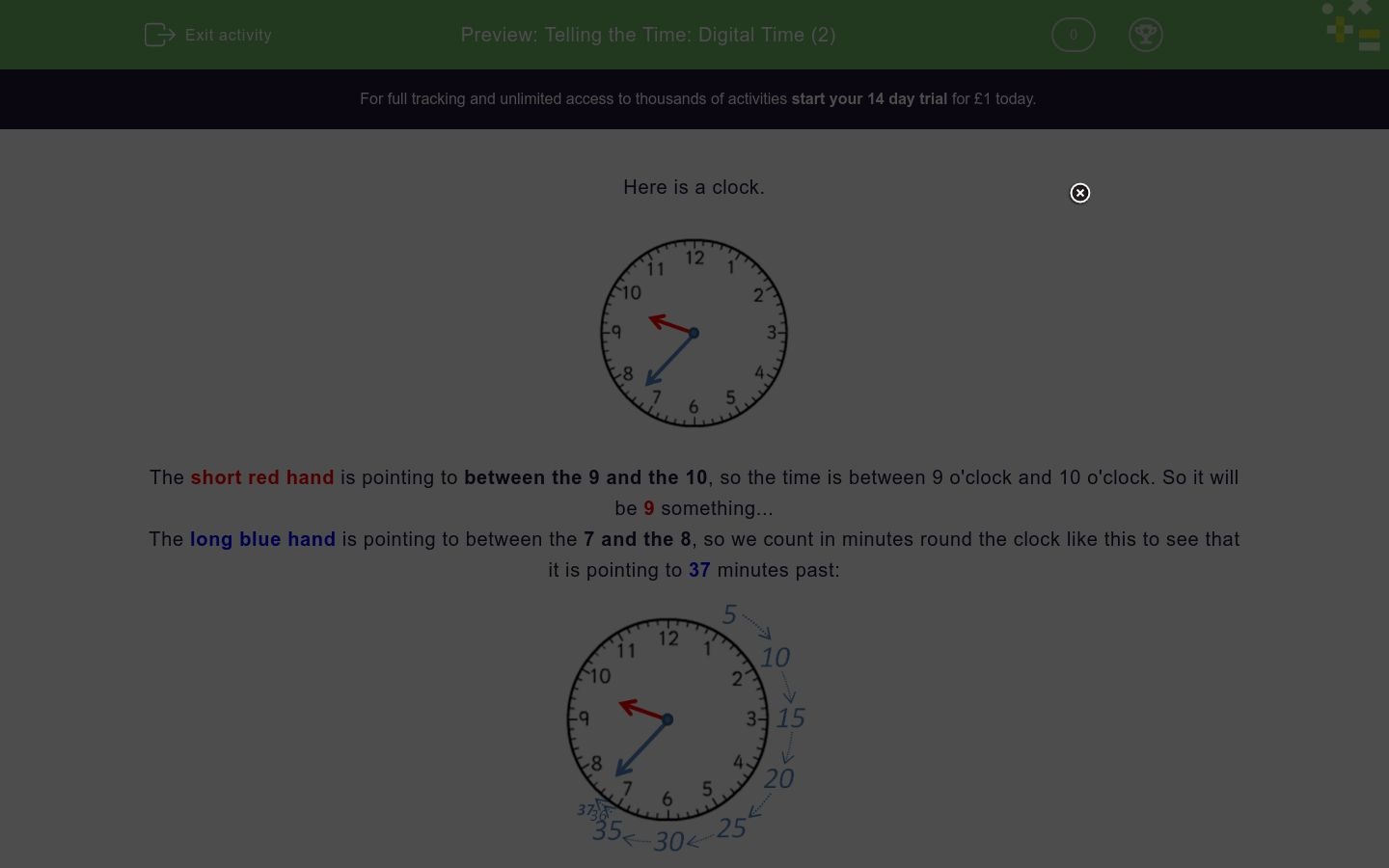Key stage:  KS 2

Curriculum topic:   Measurement

Curriculum subtopic:   Convert Analogue/Digital Time

Difficulty level:### QUESTION 1 of 10

Here is a clock.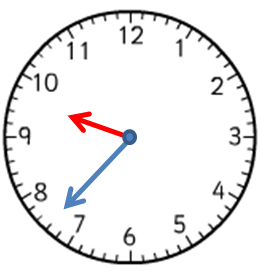The short red hand is pointing to between the 9 and the 10, so the time is between 9 o'clock and 10 o'clock. So it will be 9 something...

The long blue hand is pointing to between the 7 and the 8, so we count in minutes round the clock like this to see that it is pointing to 37 minutes past:The time shown is 9:37.

In the morning, this will be 9:37 a.m. or 09:37.

In the evening, this will be 9:37 p.m. or 21:37.

Remember

Add 12 to the hours for times after 12 noon in the 24-hour clock.

4 p.m. is 4 + 12 = 16:00

9 p.m. is 9 + 12 = 21:00

11 p.m. is 11 + 12 = 23:00 etc.

Want to understand this further and learn how this links to other topics in maths?
Why not watch this video?

The time is

9:07 p.m.

Which clock face shows this time?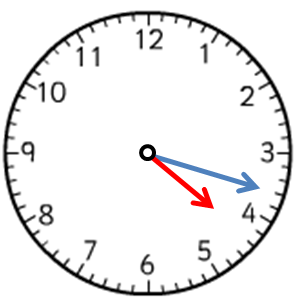A B C
A

B

C

The time is

02:30

Which clock face shows this time?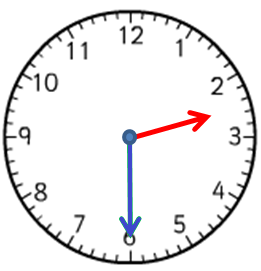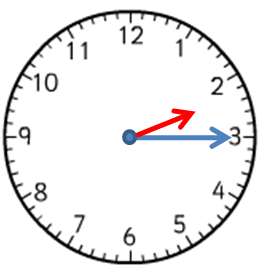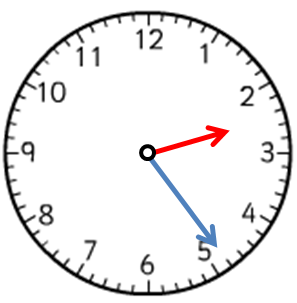A B C
A

B

C

The time is

22:37

Which clock face shows this time?A B C
A

B

C

The time is

1:54 p.m.

Which clock face shows this time?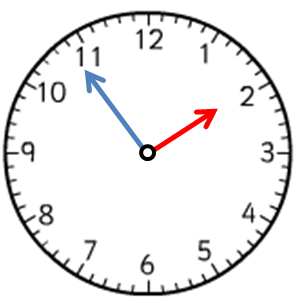A B C
A

B

C

The time is

21:46

Which clock face shows this time?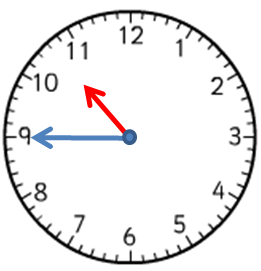A B C
A

B

C

The time is

17:12

Which clock face shows this time?A B C
A

B

C

The time is

1:25 a.m.

Which clock face shows this time?A B C
A

B

C

The time is

20:39

Which clock face shows this time?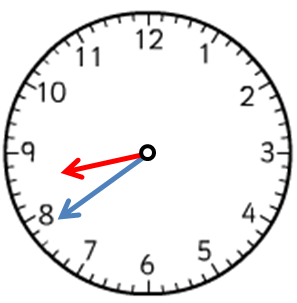A B C
A

B

C

The time is

19:51

Which clock face shows this time?A B C
A

B

C

The time is

23:58

Which clock face shows this time?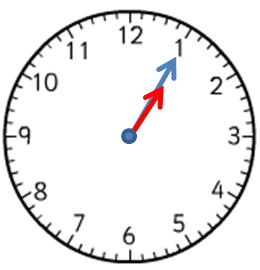A B C
A

B

C

• Question 1

The time is

9:07 p.m.

Which clock face shows this time?A B C
B
EDDIE SAYS
The short hand is pointing to between the 9 and the 10.
The long hand is pointing to 7 minutes.
• Question 2

The time is

02:30

Which clock face shows this time?A B C
A
EDDIE SAYS
The short hand is pointing to halfway between the 2 and the 3.
The long hand is pointing to the 6 or 30 minutes.
• Question 3

The time is

22:37

Which clock face shows this time?A B C
B
EDDIE SAYS
22 - 12 = 10 so this is 10:37 p.m.
The short hand is pointing to between the 10 and the 11.
The long hand is pointing to 37 minutes.
• Question 4

The time is

1:54 p.m.

Which clock face shows this time?A B C
C
EDDIE SAYS
The short hand is pointing to between the 1 and the 2, but almost at the 2.
The long hand is pointing to 54 minutes.
• Question 5

The time is

21:46

Which clock face shows this time?A B C
A
EDDIE SAYS
21 - 12 = 9 so this is 9:46 p.m.
The short hand is pointing to between the 9 and the 10.
The long hand is pointing to 46 minutes.
• Question 6

The time is

17:12

Which clock face shows this time?A B C
B
EDDIE SAYS
17 - 12 = 5 so this is 5:12 p.m.
The short hand is pointing to between the 5 and the 6.
The long hand is pointing to 12 minutes.
• Question 7

The time is

1:25 a.m.

Which clock face shows this time?A B C
B
EDDIE SAYS
The short hand is pointing to nearly halfway between the 1 and the 2.
The long hand is pointing to the 5 which is 25 minutes.
• Question 8

The time is

20:39

Which clock face shows this time?A B C
C
EDDIE SAYS
20 - 12 = 8 so this is 8:39 p.m.
The short hand is pointing to between the 8 and the 9.
The long hand is pointing to 39 minutes.
• Question 9

The time is

19:51

Which clock face shows this time?A B C
A
EDDIE SAYS
19 - 12 = 7 so this is 7:51 p.m.
The short hand is pointing to between the 7 and the 8.
The long hand is pointing to 51 minutes.
• Question 10

The time is

23:58

Which clock face shows this time?A B C
B
EDDIE SAYS
23 - 12 = 11 so this is 11:58 p.m.
The short hand is pointing to between the 11 and the 12 but very near to the 12.
The long hand is pointing to 58 minutes.
---- OR ----

Sign up for a £1 trial so you can track and measure your child's progress on this activity.

### What is EdPlace?

We're your National Curriculum aligned online education content provider helping each child succeed in English, maths and science from year 1 to GCSE. With an EdPlace account you’ll be able to track and measure progress, helping each child achieve their best. We build confidence and attainment by personalising each child’s learning at a level that suits them.

Get started